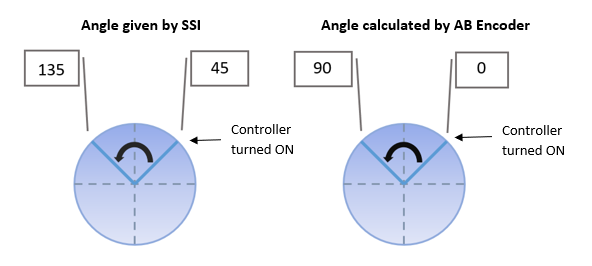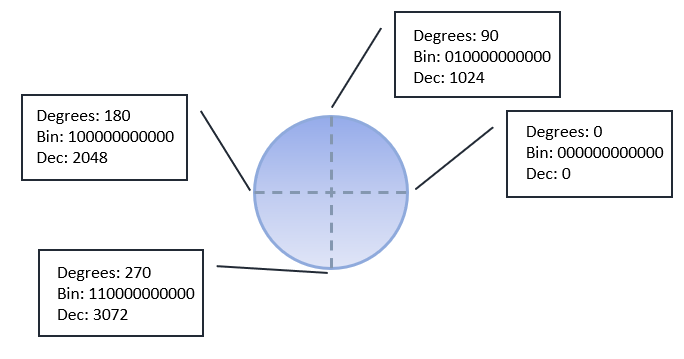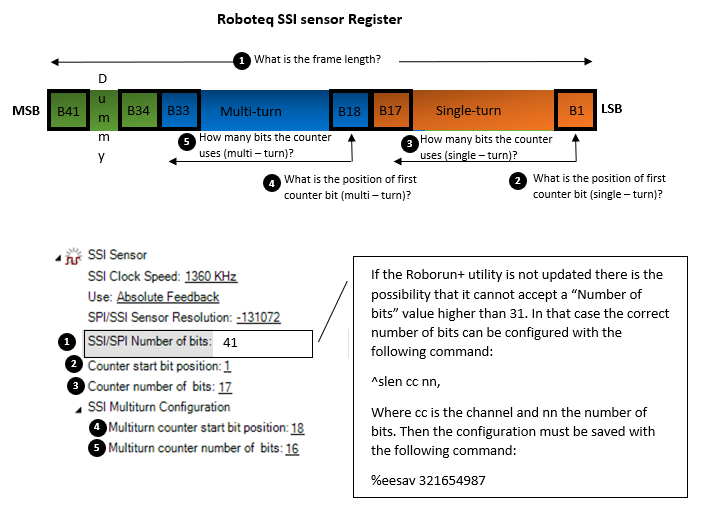SSI sensors

SSI sensors are absolute encoders that give a signal that corresponds to the shaft’s position. The term “absolute” is referring to the ability of the sensor to report anytime the exact position of the shaft without the need to have a reference point. Even if the whole system is power cycled and the shaft is moved, the sensor will be able to report the correct position. Their operation can be better understood compared to the operation of quadrature encoders. Quadrature encoders will not give the exact angle, but they will generate a number of pulses that will be relevant to the displacement of the sensor. The controller will have to measure those pulses and calculate the sensor angle, this angle though will not be absolute but relative to the initial position of the sensor.Interface

SSI sensors use Synchronous Serial Interface which is based on RS-422 standards. The data are transmitted using differential signals that provide better noise immunity and allow longer cables. The sensor will send the shaft angle encoded in a sequence of bits (data frame) and the transmission of each bit will be synched by the clock signal of the controller. The sensor will send a bit for each clock pulse that will receive until the transmission has finished. The number of clock pulses that the controller will send to read the sensor angle depend on the sensor resolution (12 bits, 14 bits etc.). The clock signal will be generated every time the controller needs to know the position of the sensor which may happen many times every 1 ms, depending on the firmware version.

Below is a simplified waveform of an 8-bit sensor data transmission (the inverted signals are not presented).Roboteq so far supports only binary SSI sensors. This refers to sensors that express the sensor angle in binary numerical system. By taking that into account, we can calculate the sensor angle in the previous example as follows:

SSI data is 10110110. This corresponds to 182 in binary (most significant bit first). Because the sensor is using 8 bits, its resolution is 255 counts per one sensor rotation. So, the angle of the sensor is (182/255)*360 = 257 degrees.

Sensor resolution

Different SSI sensors use a different number of bits to present the senor angle. The higher the number of bits, the higher the resolution of the sensor will be. So, 12-bit sensors will give 4095 counts per one full rotation, 14-bit sensors will give 16383 counts etc.  Once the sensor has performed a full cycle, the angle will reset. Below is an example of a 12-bit SSI sensor demonstrating the frames that the sensor will send at 0, 90, 180 and 270 degrees:Multi-turn operation

From what has been said so far, it is understood that the angle of the SSI sensor will reset every time the motor shaft has performed one full rotation. What happens though if the application requires to know besides of the motor angle, the number of the motor turns? Of course, the master controller could record and save the multi-turn data, but this would work only if the motor does not rotate after the system has turned off. That problem comes to solve the multi-turn SSI sensor, whose construction will allow to send both angle (single-turn data) and number of turns (multi-turn data) in the same data frame. Same as before, the reported angle will be valid even if the system power cycles and the shaft is rotated. The multi-turn angle will be increased by one every time the single-turn counter overfloats.  The maximum value that the multi-turn and single-turn angle can have depends on the multi-turn and single-turn resolution.

SSI variations

The frame structure of the SSI sensors is not being regulated by any standard, so the way that each sensor presents the data might vary. Both multi-turn and single-turn data can have different lengths and the position that they exist inside the frame can be arbitrary. Moreover, many sensors interpose data in the frame that are irrelevant to the angle (status flags, start-stop bits etc.) and that makes their interface even more challenging. Roboteq has implemented on 2.2 and 3+ firmware versions a method to define the location of multi-turn and single-turn data inside the frame so multi-turn SSI sensors can be fully supported.

Roboteq implementation

The controller will read the full SSI frame and then will extract the multi-turn and single-turn angle. The single-turn angle will be used for the commutation of the motor and the multiturn together with the single turn angle will be used for feedback. The feedback counter’s value will be:

Multiturn_counter * singleturn_counter + singleturn_counter ' Multiturn_counter*angle_max_val+angle

Note that although the controller will support SSI multiturn sensors with a frame length up to 47 bits, the feedback counter is 32 bits, so it can have a maximum decimal value of +- 2,147,483,647.

The controller can be configured by using Roborun+ utility to read any SSI sensor by using as a reference the sensor’s data frame that can be found on the sensor’s datasheet.

Roborun+ configuration

Below there is an example showing how to configure a 41-bit multiturn SSI sensor that Nidec motors are using. Its data frame consists of 41 bits, of which the 8 first are dummy (are all low) and the rest 33 carry the position value. Of those 33 bits the first 16 correspond to the multi-turn angle and the last 17 bits to the single-turn angle.The controller will read all 41 bits and save them on a register. The first bit of the register will be the bit of the frame that will be received last, which in that example corresponds to the less significant bit of the single turn data. The information that the controller will require are shown below: